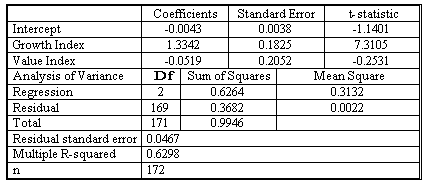### CFA Practice Question

There are 364 practice questions for this topic.

### CFA Practice Question

Suppose you want to know whether Fidelity Select Technology Fund (FSTF) behaves more like a large-cap growth fund or a large-cap value fund. You want to estimate the regression Y-hatt = b0 + b1 X1t + b2 X2t + et, where Y-hatt is the monthly return to the FSTF, X1t is the monthly return to the S&P 500 Growth Index, and X2t is the monthly return to the S&P 500 Value index. The table below shows the results of a multiple linear regression using monthly data from December 1994 through March 2009.Determine whether both the monthly return to the growth index and the monthly return to the value index jointly are statistically related to the monthly return to the FSTF at the 5% level of significance.
Correct Answer: To conduct the F-test, we need four inputs:
• Total number of observations (n): 172
• Total number of parameters to be estimated (k + 1): 3
• Sum of squared errors or residuals, abbreviated as SSE: 0.3682.
• Regress sum of squares: 0.6264.
F = [0.6264/2] /[0.3682/(172 - 3)] = 143.75.

The large value of F indicates that the null hypothesis is false, so we can reject at the 5% level that the slope coefficients are all 0.

User Comment
hrai123 F statistic= MS regression/MS residual
katybo F 0,05 (2,169) = 3 < 143,75
MasterD Confirmed:
Excel F Critical is:
FINV(0.05,2,169) = 3.0494
Table Lookup F(0.05,2,infinity) = 3.00

Also, if you used the rounded values of
0.3132 / .0022 = 143.3636

3.0494 < 143.3636 thus we can reject the null hypothesis
ericczhang MSR and MSE are already reported right besides the 'Sum of Squares' column so you could just use those directly.
sahilb7 @ericczhang Exactly. The solution is given here is more complicated.